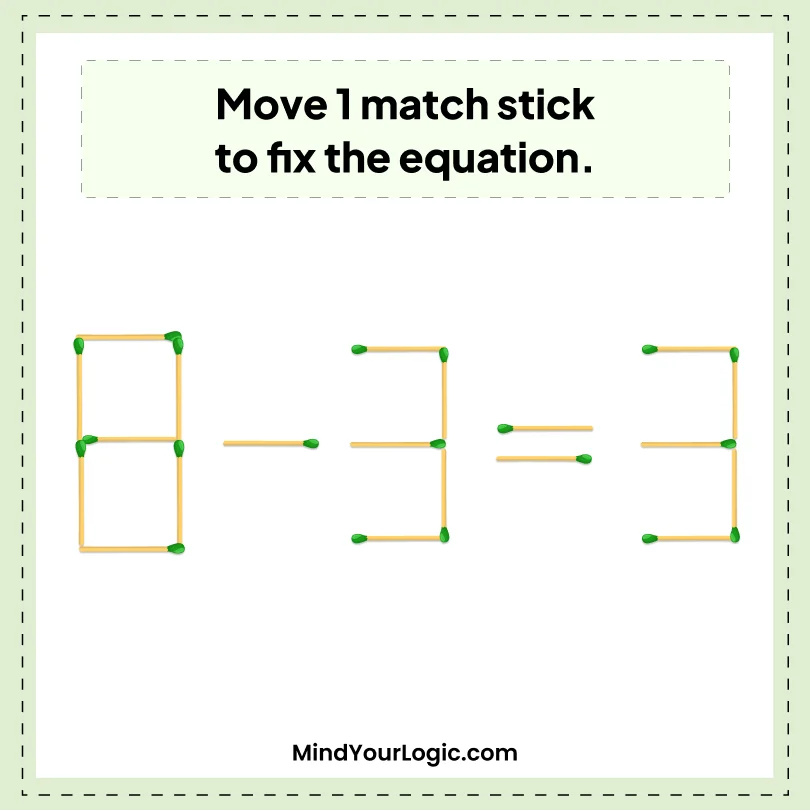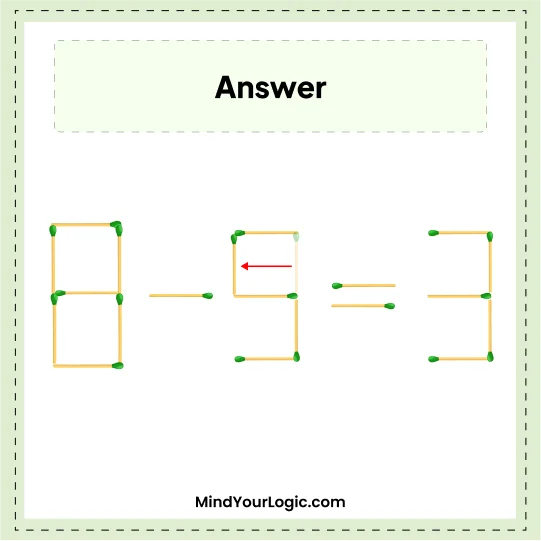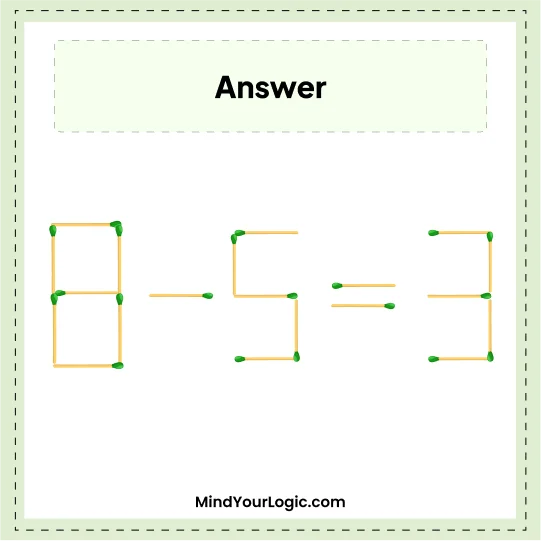# 8-3=3 - Matchstick Equation

###### 40.Matchstick Puzzles
`Move one matchstick and fix the equation.`•

Explanation :

``` Move one stick from 3 and make it 5.
The equation will look like 8 - 5 = 3.```